# Non Verbal Reasoning - Dot Situation

### Exercise :: Dot Situation - Section 1

From amongst the figures marked (1), (2), (3) and (4), select the figure which satisfies the same conditions of placement of the dots as in figure (X).

6.

Select the figure which satisfies the same conditions of placement of the dots as in Figure-X.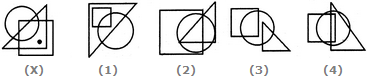A. 1 B. 2 C. 3 D. 4

Explanation:

In fig. (X), the dot is contained in the region common to the triangle and the square only. Out of the four alternatives, only fig. (1) contains a region common to the triangle and the square only.

7.

Select the figure which satisfies the same conditions of placement of the dots as in Figure-X.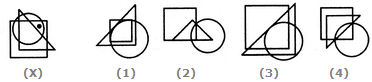A. 1 B. 2 C. 3 D. 4

Explanation:

In fig. (X), the dot is contained in the region common to the square and the circle only. Out of the four alternatives, only fig. (4) contains a region common to the square and the circle only.

8.

Select the figure which satisfies the same conditions of placement of the dots as in Figure-X.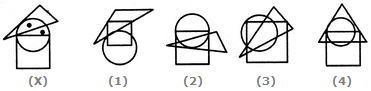A. 1 B. 2 C. 3 D. 4

Explanation:

In fig. (X), one of the dots lies in the region common to the circle and the triangle only and the other dot lies in the circle alone. In each of the two figures (1) and (2), there is no region common to the circle and the triangle only. In fig. (4) there is no region which lies in the circle alone. Only fig. (3) consists of both the types of regions.

9.

Select the figure which satisfies the same conditions of placement of the dots as in Figure-X.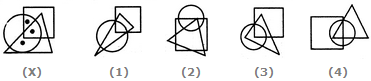A. 1 B. 2 C. 3 D. 4

Explanation:

In fig. (X), one of the dots lies in the region common to the circle and the triangle only, another dot lies in the region common to all the three figures - the circle, the square and the triangle and the third dot lies in the region common to the circle and the square only. In each of the figures (1) and (3), there is no region common to the circle and the square only and in fig. (2), there is no region common to the circle and the triangle only. Only fig. (4) consists of all the three types of regions.

10.

Select the figure which satisfies the same conditions of placement of the dots as in Figure-X.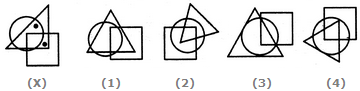A. 1 B. 2 C. 3 D. 4